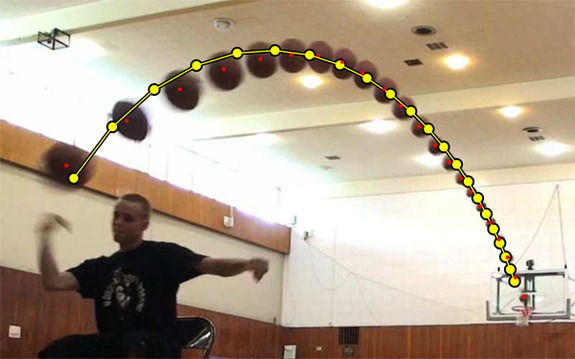top of page

## The Laws of Motion

​          Newton’s First Law states that an object at rest or in motion will remain unless the object experiences a net external force. Inertia is the tendency of an object to maintain its current state or the tendency not to accelerate. A baseball (low mass) accelerates more than a softball (high mass) if the same force is applied to both. Thus, it requires less force to accelerate a low mass object than it does to accelerate a high mass object.

Newton's Second Law of Motion relates force, mass, and acceleration. It states that the acceleration of an object is directly proportional to the net force acting on the object and inversely proportional to the object's mass (acceleration = force/mass). An object will exert a force on another object that interacts with it in its environment. Forces always exist in pairs. Newton described this type of situation with his third law of motion.

Newton's Third Law states for every action, there is an equal and opposite reaction. Projectiles are objects that are under the sole influence of gravity. They represent a form of two dimensional or projectile motion. External ballistics involves the forces on a bullet from the time it exits the barrel of a gun until it hits its target. When a bullet exits a weapon, the force created due to the energy produced upon igniting the solid gunpowder in the combustion chamber, causes the material to combust into a gaseous state - converting the stored chemical energy into kinetic energy of motion, which then becomes acted upon by an opposing force, gravity. Gravity will pull the bullet/projectile into a downward motion. Neglecting air resistance, a projectile has a constant horizontal velocity and a constant downward free-fall acceleration. In the presence of air resistance, projectiles follow a parabolic path or trajectory as shown in the image below.

Image Source: FourandSix technologies - The parabolic projectile motion of an object fired from a cannon under the influence of gravity and air resistance

Before the ball is thrown, the ball is at rest. When the ball is thrown, kinetic energy yields a force to push the ball forward. After the ball is released, it travels in a straight line until impacted upon by gravity and wind resistance. The ball will have a velocity (change in distance with change in time); it will also accelerate (change in velocity with change in time). Maximum acceleration would be right as ball leaves the fingertips of the player. The acceleration of the ball is directly proportional to the force applied due to the kinetic energy and indirectly proportional to the mass of the ball. The ball will now have a parabolic path downward towards the goal. When the ball hits either the backboard or the enters the net (swoosh!), energy will be transferred to those objects. An air ball will transfer much of its energy to the floor, a brick shot will transfer its energy to the backboard, and a net shot will transfer some of its energy to the net and the remaining will hit the floor. Remember, the ball will bounce due to it exerting an equal and opposite force on the ground and the ground exerts a force on the ball which will eventually stop the ball due to floor friction.bottom of page